Numerical Simulation of Thermomechanical Problems in Applied Mechanics: Application to Solidification Problem

Vincent Obiajulu OGWUAGWU

Mechanical Engineering Department, Federal University of Technology, Minna, Nigeria

Abstract

Thermomechanical coupling problems are common phenomena in the field of Solid Mechanics. In these problems, the mechanical response of such solids depends on the thermal behaviour of the medium and vice versa. The present study presents the two-dimensional numerical simulation of stress distribution in a cooling ingot during continuous casting of steel. Results obtained from the simulation show good representation of stress distribution in ingot cooling and compares well with experimental results from past studies.

Keywords

Thermomechanical coupling; Ingot cooling; Temperature stresses; Phase transformation

Introduction

Many problems exist in the physical sciences in which there exist coupling between mechanical and thermal phenomena. Increase in the temperature of a body can lead to changes in deformation behaviour of the body which in turn may lead to variation in stresses (commonly referred to as temperature stresses). This is a common phenomenon in the solidification of metals in moulds as well as cooling of welds etc. In general, the mechanical response of such bodies depends on their thermal behaviour.

In this paper, a thermomechanical problem involving the solidification and cooling of steel ingot is presented. Numerical analysis of material processing is usually very complicated due to coupling among the temperature field, stress-strain history and the material phase transformations (-).

Mathematical Modelling

Figure 1 shows a schematic diagram of the coupling between temperature, stress-strain and phase transformation.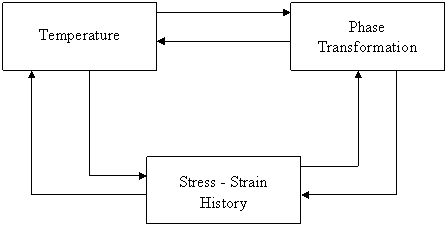Figure 1. Coupling between temperature, stress-strain and phase transformation

Coupling between temperature and phase transformation

Materials phase transformations are basically governed by the temperature profile, as indicated in path 1 in figure 1. For slow change in temperature, the equilibrium phase diagram can be used to describe the phase transformation. In this case, only the temperature determines the material constitution. For fast cooling rates, however, the continuous cooling transformation is most appropriate.

The temperature field can be affected by the phase transformation through the release or absorption of the latent heat of transformation as shown by path 2 in figure 1. The latent heat effect is basically a heat increase given by the relation:(1)

where k is the thermal conductivity, a is the thermal expansion coefficient, q is the internal heat generation rate, ev is the elastic volume strain, Sij is the deviatory stress components and eijp is the plastic strain component. The third term on the right hand side of equation (1) represents the elastic expansion work while the last term is the energy dissipation due to plastic deformation.

Coupling between temperature and stress-strain histories

The change of temperature field causes thermal strain, represented by path 3 in figure 1. Also, the stress-strain histories can affect the temperature due to the work of volume change and plastic deformation. Generally, these terms usually have little effects on the temperature filed for small deformations.

Coupling between transformation and stress-strain histories

The total strain can be divided into several components given by: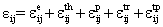(2)

The phase transformation includes a transformation strain eijtr in equation (2) above. This is usually caused by the difference of the specific volume of the phases involved in the phase change. There is also transformation plasticity eijtp caused by inelastic deformation even at stresses below yield strength. The change in volume fractions during phase transformation leads to change in mechanical properties as indicated by path 4 in figure 1.

The elastic strain and stress are related by the following constitutive equation: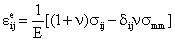(3)

To obtain the incremental strain, we subtract all the other strain components from the total strain increment as: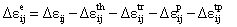(4)

E and n are both temperature and material composition dependent. For n coexisting phases, the following relations hold: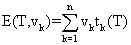,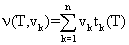(5)

where the subscript is the number of the phase and vk is the volume fraction of the phase.

The total thermal strain is given as: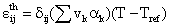(6)

In the rate form, equation (6) can be written in the form: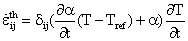(7)

where a is the average values of the thermal expansion coefficient given by: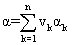(8)

Generally, a mean value of a from a reference temperature to any temperature within the range of interest can be used to calculate the thermal strain, which is defined as: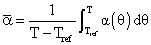(9)

The thermal strain increment is calculated as: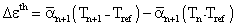(10)

which account for change in thermal strain in a time increment.

For phase transformation involving a number of phases, the transformation strains for an isotropic material is given by: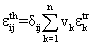(11)

The transformation plasticity can be determined from the total transformation plastic strain formulation as: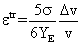(12)

where YE is the yield strength of the weaker of the two phases involved and Dv/v is the volume strain.

The rate formulation is given as: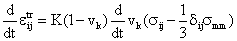(13)

The von Mises yield criterion is used with linear isotropic hardening rule for the plastic strain.

Now, the stress model for two-dimensional plane stress problems is derived from the equation of equilibrium given as: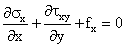,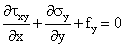(14)

where the relation between strain and displacement are given by: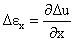,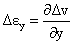,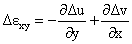(15)

which in matrix form becomes:

Δe = BΔu                                                                                                        (16)

where B is the matrix defined as:

B =(17)

Utilizing the principle of virtual work for equilibrium conditions gives: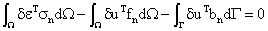(18)

Then for small increments Ds, Db, and Df the equilibrium condition still holds and equation (22) is still satisfied. The finite element discretion for displacement and strain are:

u = ΣNidi-NTd, δu = NTδd, e = ΣBidi-BTd; δe = Bδd

which on substitution into equation (18) yields: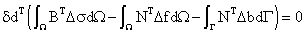(19)

Or since equation (19) is satisfied for arbitrary values of dd, we obtain: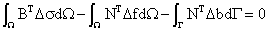(20)

Also, for isotropic materials, equation (19) is given in matrix form as:

Δσ = D1Δe + D2ΩT

which on substitution into equation (20) yields:

KΔd = ΔR = ΔRth + ΔRm                                                                                 (21)

where:

K = ∫ΩBTD1BdΩ

ΔRth = ∫ΩBTD2ΔTdΩ

ΔRm = ∫ΩNTΔfBdΩ + ∫ΓNTΔfBdΓ

Results and Discussions

From the stress histories of figures 3 and 4 respectively, the effect of phase transformation zone can be traced. The outer surfaces express tensile stresses immediately after solidification and then reversal to compression. The symmetrical axes of the cast are subjected to tensile stress state after complete solidification as expected.

The temperature contours and principal stresses along the axis of symmetry and at the outer surfaces are shown in Figures 2, 3, 4, and 5 respectively.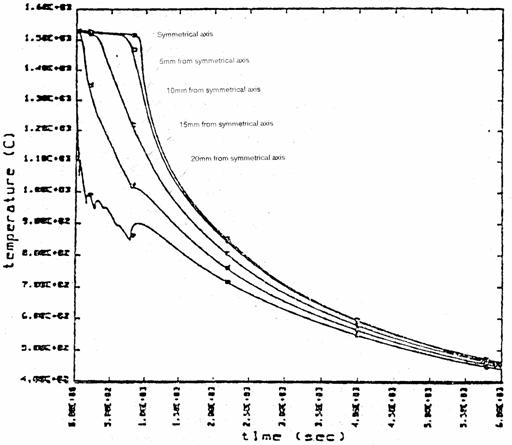Figure 2. Temperature histories along the axis of symmetry

The cast completely solidified after about 900 seconds. The liquids core of the cast gave rise to small tensile stresses while the outer solid shell is in compression. The high transient tensile stress at the surface combined with some other solidification factors may cause some defects. Unloading to an elastic state caused a corresponding deep gradient in the stress field. These unloading conditions corresponding to the stress reversal are evident in figures 3 and 4.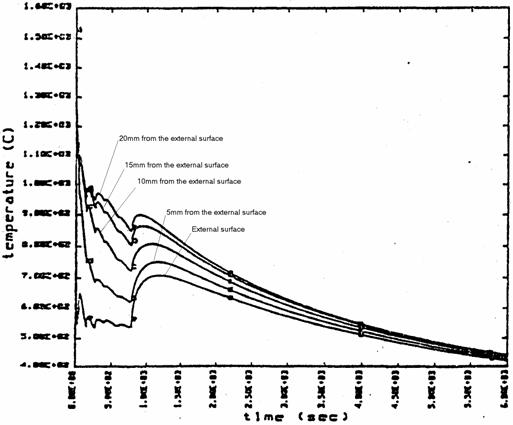Figure 3. Temperature histories at the outer surface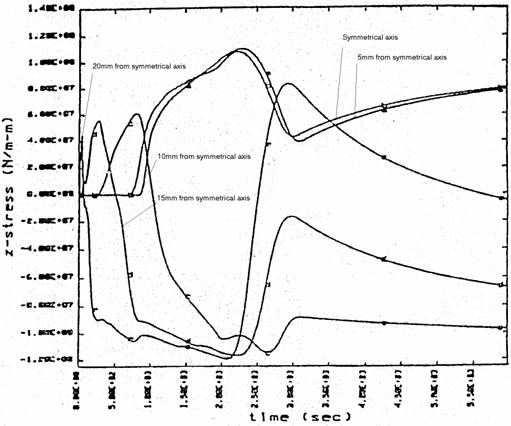Figure 4. Stress histories along the axis of symmetry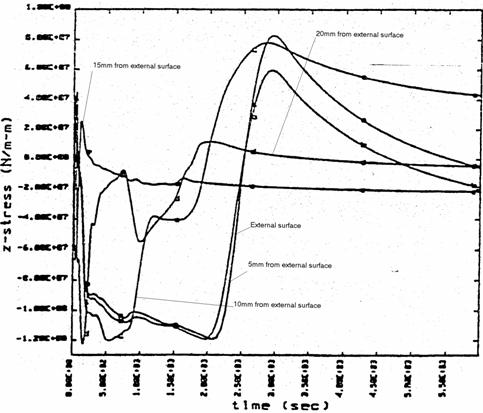Figure 5. Stress histories at the outer surface

Based on the information obtained from the developed principal stresses, it can be predicted if a given process conditions would lead to some defects such as cracks. For instance, if the maximum principal stress at the centre of the casting exceeds some criteria for crack formation, then at that temperature, there may be the possibility of initiation and propagation of longitudinal cracks. This is as a result of the random orientation of the grains at the centre.

References

 Inoue T., Wang Z., Coupling between Stresses, Temperature, and Metallic Structure During Processes Involving Phase Transformations, Materials Science Technology, 1, p. 845-850, 1985.

 Sjostrom S., Interactions and Constitutive Models for Calculating Quench Stresses in Steel, Material Science and Technology, 1, p. 823-829, 1985.

 Wang Z. Inoue T., Viscoplastic Constitutive Relation Incorporating Phase Transformation: Application to Welding, Material Science and Technology, 1, p. 899-903, 1985.

 Vaz Jr. M. Owen D. R. J., Thermo-mechanical Coupling and Large Scale Elasto-plastic Problems, Application of Numerical Methods in Engineering, 1997.